# I tried using 'Wolfram Alpha' which solves difficult mathematical expressions in one shot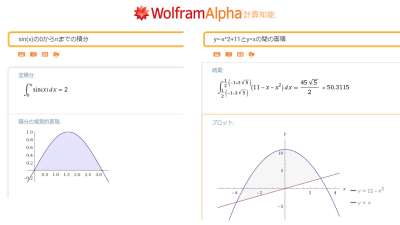Wolfram Alpha is a web service that answers mathematical formulas entered in the form and various questions, and it is operated by Wolfram Research , known as the developer of scientific computing software " Mathematica ". This service is being offered Japanese language service on Monday, June 18, 2018, and at the time of article creation, it corresponds only to questions concerning mathematics. I actually tried using what it is like.

Wolfram | Alpha: Computational Intelligence
http://en.wolframalpha.com/

When you access Wolfram Alpha , the input form is displayed.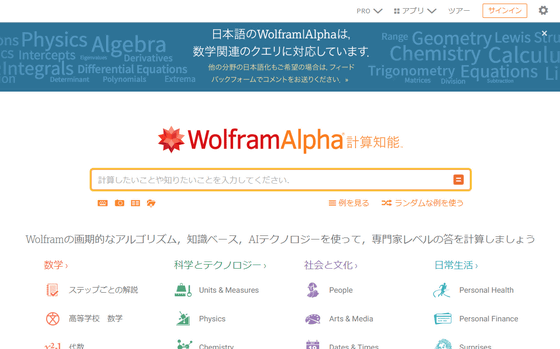Immediately input a simple linear equation "y = 2x + 1" that somehow came up with and click "=".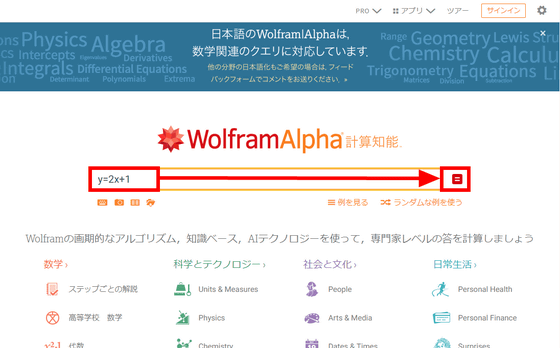Then, detailed information of y = 2x + 1 is displayed and the formula input to "input", the shape when graphing it into "geometric figure", the graph in "plot", the graph on the right side You can check the mathematical formulas, roots, characteristics of real functions, and values ​​when partial differentiation is done to set 0 to 0.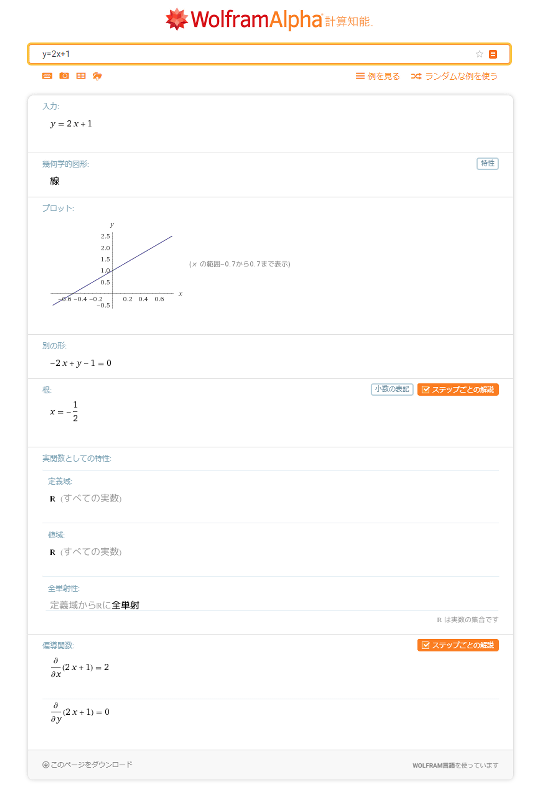Here, when you click "Properties" in the "Geometric figure" column showing the shape when graphing ...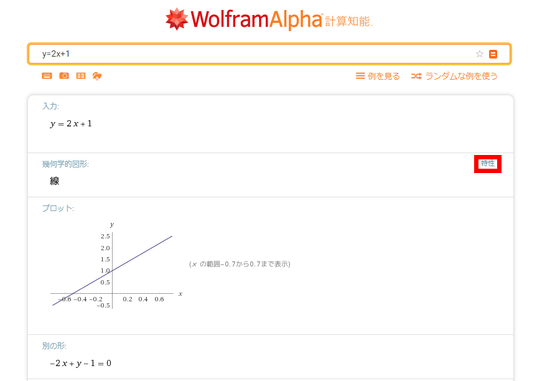The values, slope, curvature of intercepts showing the intersection with the x and y axes are displayed.

Also, when you click "Decimal notation" in "Roots" ... ...

You can switch the value of the root from fraction to decimal. Also, click "Commentary for each step" in the upper right ... ...

On the way to derive roots, commentary at the beginning is displayed. It seems necessary to register for paid service of Wolfram Alpha in order to display all commentary.

Next let's display trigonometric function sin. Enter "sin (x)" on the input form and click "=" ......

Detailed information was displayed more than the first-order function.

Wolfram Alpha supports Japanese as well as mathematical expressions. Try typing " Tower of Hanoi " on the input form ... ...

Move the discs one by one according to the rules, and solve the "Tower of Hanoi" puzzle which moves all the discs to the right edge of the picture was displayed one step at a time.

The initial setting of Hanoi's tower displayed by Wolfram Alpha is 4, but changing the number of disks to 5 and clicking "=" ... ...

The solution of 5 disc Hanoi towers is displayed, you can see that it can be solved in 31 steps in total.

In addition, " Fourier series " ... ...

Taylor expansion and other advanced features also supported. It is perfect for those studying mathematics.

Furthermore, on the input form of Wolfram Alpha, letting the wording such as "the area between y = - x ^ 2 + 11 and y = x" be input to calculate the value of the area surrounded by the two functions It also supports such as, Calculator solves problems that are difficult to calculate with a single shot.

Although it is possible to use it for free if it is within the range you have used so far, Wolfram Alpha also has a paid plan. When registering in the "Pro" plan of 780 yen per month, "Commentary for each step" "Practice problem" "Analysis function by uploading files up to 2 MB" "Extension of calculation time" "Function to make display results more graphical" "Finance , Nutritional web application "and so on will be available. In addition, the "Pro Premium" plan of 1350 yen per month is that in addition to the contents of the Pro plan, "analysis function by uploading files up to 5 MB", customer support etc will be available.

in Review,   Web Service, Posted by darkhorse_log